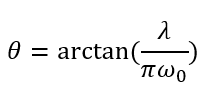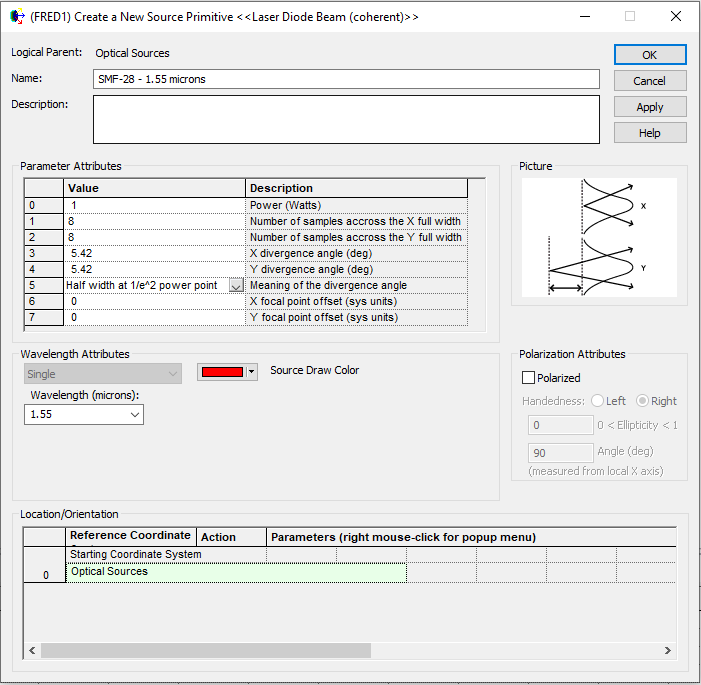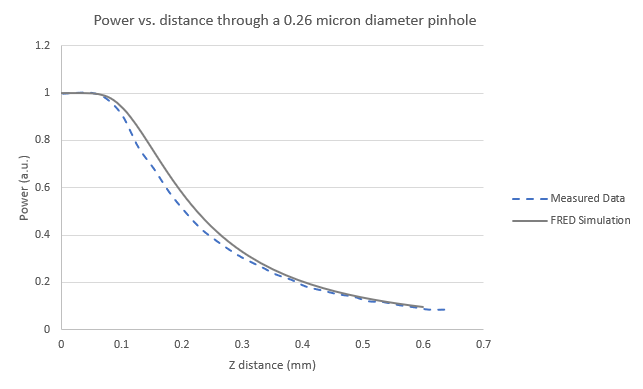# Single mode fiber output - Coherent Source

The optical field exiting a single-mode fiber can be well described by constructing a Gaussian beam at the fiber exit face whose 1/e2 diameter matches the mode-field diameter of the fiber (AM Kowalevicz, Beam Divergence from an SMF-28 Optical Fiber, 2006).  This KB article demonstrates how to configure a Laser Diode Beam (coherent) type Source Primitive to represent the optical field leaving a Corning SMF-28 single mode fiber.

At 1.55 microns, the mode-field diameter of SMF-28 is 10.4 microns.  However, the Laser Diode Beam (coherent) type Source Primitive in FRED is specified by its far-field divergence angle rather than the beam waist size.  For a Gaussian beam, the relationship between divergence angle, theta, and beam waist radius, w, is given by the equation below.Using 5.2 microns as the beam waist radius, w0, and 1.55 microns as the beam wavelength, lambda, the far-field divergence angle of the Gaussian beam is calculated to be 5.42 degrees.  In the Laser Diode Beam source dialog, the far-field beam divergence angle is entered into parameters 3 and 4 since the beam is symmetric.  Parameter 5 of the source construction defines the interpretation of the angle value, which should be set as "Half width at 1/e2 power point", since we used the fiber mode radius (not diameter) to calculate the divergence angle.  Lastly, a wavelength value of 1.55 microns is entered in the wavelength attributes portion of the dialog.In the Laser Diode Beam source model construction above, parameters 1 and 2 control the angular sampling of the coherent field being described and should be adjusted in powers of 2 as needed to improve the accuracy of the beam representation.  The required sampling is dependent on the system model that the fiber source is being launched into but, generally, higher sampling values result in improved accuracy in the field calculation.

The example FRED file below defines an SMF-28 fiber output source using the procedure described above.  It also contains an analysis surface and an embedded script that can be used to duplicate the results of the Pinhole Power Measurement section in the Kowalevicz paper referenced above.  A comparison between the FRED pinhole simulation results and the measurement data from the Kowalevicz reference paper (figure 10) are shown below.  The deviation between the Gaussian beam representation and the measured data agree with the same calculation performed by Kowalevicz.Example FRED File: SMF28.frd